HSPT Math : Problem Solving

Example Questions

1 2 53 54 55 56 57 58 59 61 Next →

Example Question #601 : Problem Solving

A certain cube has a side length of 25 m.  How many square tiles, each with an area of 5 m2, are needed to fully cover the surface of the cube?

500

750

100

200

1000

750

Explanation:

A cube with a side length of 25m has a surface area of:

25m * 25m * 6 = 3,750 m2

(The surface area of a cube is equal to the area of one face of the cube multiplied by 6 sides. In other words, if the side of a cube is s, then the surface area of the cube is 6s2.)

Each square tile has an area of 5 m2.

Therefore, the total number of square tiles needed to fully cover the surface of the cube is:

3,750m2/5m= 750

Note: the volume of a cube with side length s is equal to s3.  Therefore, if asked how many mini-cubes with side length n are needed to fill the original cube, the answer would be:

s3/n3

Example Question #1 : How To Find The Surface Area Of A Cube

A company wants to build a cubical room around a cone so that the cone's height and diameter are 3 inch less than the dimensions of the cube. If the volume of the cone is 486π ft3, what is the surface area of the cube?

486 in2

69,984 in2

726 in2

73,926 in2

513.375 in2

73,926 in2

Explanation:

To begin, we need to solve for the dimensions of the cone.

The basic form for the volume of a cone is: V = (1/3)πr2h

Using our data, we know that h = 2r because the height of the cone matches its diameter (based on the prompt).

486π  = (1/3)πr* 2r = (2/3)πr3

Multiply both sides by (3/2π): 729 = r3

Take the cube root of both sides: r = 9

Note that this is in feet. The answers are in square inches. Therefore, convert your units to inches: 9 * 12 = 108, then add 3 inches to this: 108 + 3 = 111 inches.

The surface area of the cube is defined by: A = 6 * s2, or for our data, A = 6 * 1112 = 73,926 in2

Example Question #601 : Problem Solving

Angie is painting a 2 foot cube for a play she is in. She needs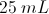of paint for every square foot she paints. How much paint does she need?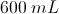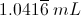It is impossible to convert between metric units and feet.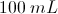Explanation:

First we must calculate the surface area of the cube. We know that there are six surfaces and each surface has the same area: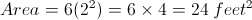Now we will determine the amount of paint needed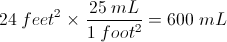Example Question #1 : How To Find The Surface Area Of A Sphere

A spherical orange fits snugly inside a small cubical box such that each of the six walls of the box just barely touches the surface of the orange.  If the volume of the box is 64 cubic inches, what is the surface area of the orange in square inches?

256π

128π

64π

32π

16π

16π

Explanation:

The volume of a cube is found by V = s3.  Since V = 64, s = 4.  The side of the cube is the same as the diameter of the sphere.  Since d = 4, r = 2.  The surface area of a sphere is found by SA = 4π(r2) = 4π(22) = 16π.

Example Question #1 : How To Find The Area Of A Rectangle

Steve's bedroom measures 20' by 18' by 8' high. He wants to paint the ceiling and all four walls using a paint that gets 360 square feet of coverage per gallon. A one-gallon can of the paint Steve wants costs $36.00; a one-quart can costs$13.00. What is the least amount of money that Steve can expect to spend on paint in order to paint his room?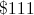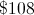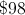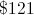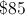Explanation:

Two of the walls have area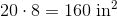; two have area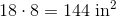; the ceiling has area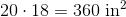Therefore, the total area Steve wants to cover is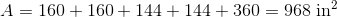Divide 968 by 360 to get the number of gallons Steve needs to paint his bedroom: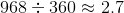Since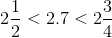, Steve needs to purchase either two gallon cans and three quart cans, or three gallon cans.

The first option will cost him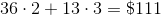; the second option will cost him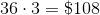. The latter is the more economical option.

Example Question #604 : Problem Solving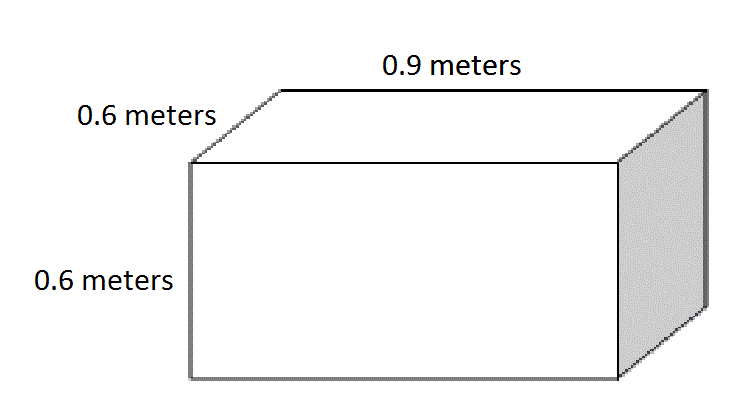Give the surface area of the above box in square centimeters.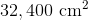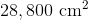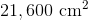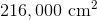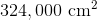Explanation:

100 centimeters make one meter, so convert each of the dimensions of the box by multiplying by 100.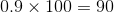centimeters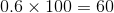centimeters

Use the surface area formula, substituting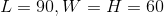: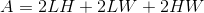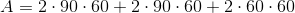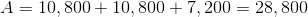square centimeters

Example Question #601 : Problem Solving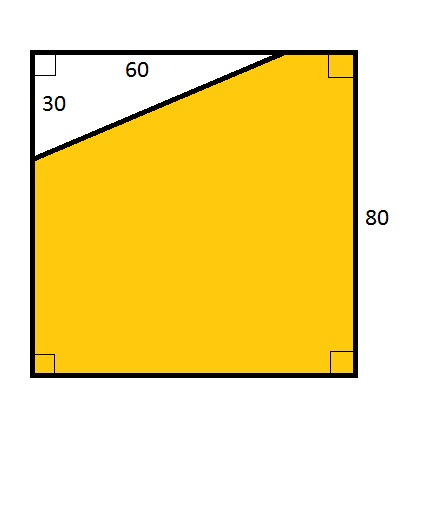Note: Figure NOT drawn to scale.

Refer to the above diagram, which shows a square. Give the ratio of the area of the yellow region to that of the white region.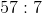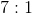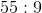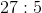The correct answer is not given among the other choices.Explanation:

The area of the entire square is the square of the length of a side, or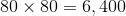.

The area of the right triangle is half the product of its legs, or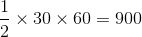.

The area of the yellow region is therefore the difference of the two, or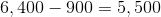.

The ratio of the area of the yellow region to that of the white region is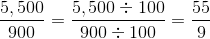; that is, 55 to 9.

Example Question #121 : Plane Geometry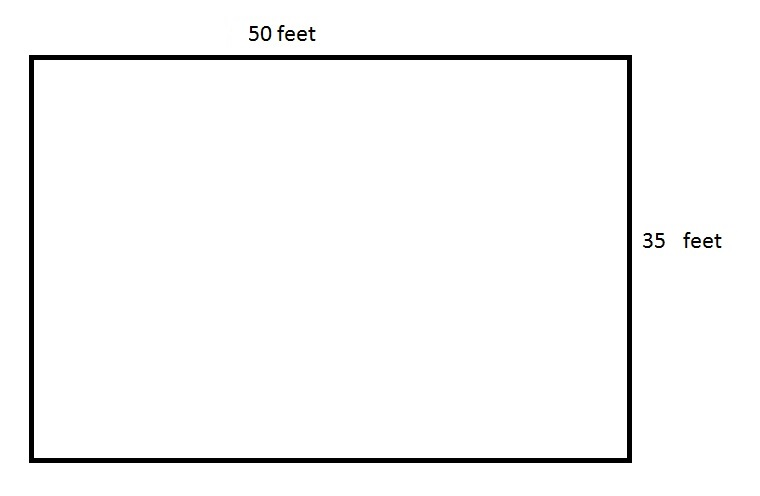The above depicts a rectangular swimming pool for an apartment. The pool is five feet deep everywhere.

An apartment manager wants to paint the four sides and the bottom of the swimming pool. One one-gallon can of the paint he wants to use covers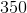square feet. How many cans of the paint will the manager need to buy?Explanation:

The bottom of the swimming pool has area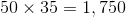square feet.

There are two sides whose area is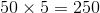square feet,

and two sides whose area is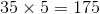square feet.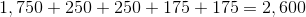square feet.

One one-gallon can of paint covers 350 square feet, so divide: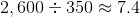Seven full gallons and part of another are required, so eight is the correct answer.

Example Question #601 : Problem Solving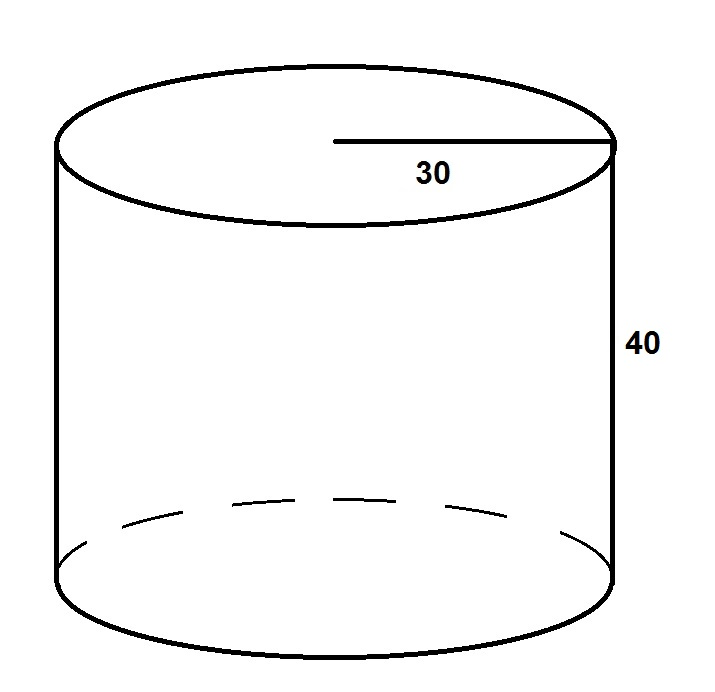The surface area of the above cylinder is 80% of what number?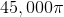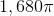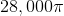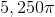Explanation:

The surface area of the cylinder can be calculated by setting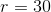and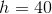in the formula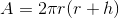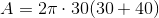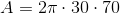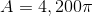This is 80% of the number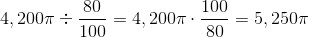Example Question #608 : Problem Solving

What is the surface area of a cube with a side edge of 7?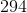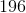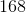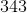Explanation:

First you must find the area of one side of the cube which is side squared.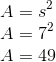.

Then you multiply that area by six since that is how many sides a cube has.

Your answer is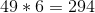.

1 2 53 54 55 56 57 58 59 61 Next →